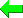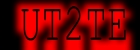SudokuWiki.org
Strategies for Popular Number Puzzles

# Sudoku String Definitions

As of 18th January 2023 I'm employing a new Sudoku string definition to transport information about the board and candidates. This is currently employed on the
• [Email this Board] feature - best place to copy/paste the new link
• The [Import a Sudoku] will accept the new 162 character string as well as the normal 81
• The transport of the solver board to the Sudoku Player does so using the new definition.
• I will probably change some of the printable pages to use this soon.
• As an example of it's usefulness I have changed the "Load example" links on the X-Wing page to precisely go to the correct position
Thus the new link looks like this example:
https://www.sudokuwiki.org/sudoku.htm?bd=vu4804032o1508g080030k10o888882647046888g06828060k10029k260b90478go003g0g95090b0b6a2c00g0bcg038ga8g9ao1506601oh847063a2ag288gg8g0626c082c2k20b159818920k9ag9462044

Users of the solver may want to know more about this string so that they can duplicate it for their own uses and understand how it works. So I'm presenting the information here.

The normal 81 character sudoku definition remains the same as before in all places and uses.

The new 162 character string takes each cell on the board and converts the clue or solution to a bit (1=1, 2=2, 3=4, 4=8, 5=16, 6=32, 7=64, 8=128 and 9=256). This allows a spread of candidates to be summed as a set of bit values. To really pack the information numbers are converted to base 32. The twist with the new definition is I wanted to also transport a flag to state if a number was a clue or solved cell. This is done by shifting the bits by one and setting the last bit as 1 for clue, 0 for not.

A bit of javascript code shows how this is done
`for (y = 0; y < 9; y++)	for (x = 0; x < 9; x++) {		n = get the cell value or set of candidates as bits		n = (n << 1) + (clue(y, x) ? 1 : 0); //  shift to make room for one more bit		h = n.toString(32); // convert to base 32		if (h.length < 2) h = '0' + h; //  pad the number if not two digits		s += h; // append to string being made	}`

To unpack a string we do the following
```// This splits a string into an array of elements each 2 characters long
var narr = theString.match(/.{1,2}/g);
for (y = 0; y < 9; y++)
for (x = 0; x < 9; x++) {
n = parseInt(narr[j * 9 + i], 32); // convert base 32 to decimal
clue(y, x) = (n & 1) ? 1 : 0; // extract the clue flag
n = n >> 1; Shift the bits to remove the flag
if (bit_count(n) == 1) {
// save the number as a clue or solution
} else {
// save the number as a set of candidates
}
}
```

The largest possible value in a cell, the sum of all numbers 1 to 9, is 511. Shifted left one bit this becomes 1022. Add the clue flag to make 1023. Converted to base 32 this becomes "vu". So no cell value will exceed 2 "digits" in this scheme. Hence it makes sense to use a fixed length definition rather than one with delimiters.

Any problems or questions, let me know in the comments below

 Please enter theletters you see:Remember me